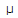# Electronics - Quantities and Units

Exercise : Quantities and Units - Filling the Blanks
1.
7450 × 10–5 amperes equals 74.5 ___.
A
mAA
nA
Explanation:
No answer description is available. Let's discuss.

2.
The number 5200 ×10–2 equals ___ × 101.
0.52
5.2
52
520
Explanation:
No answer description is available. Let's discuss.

3.
The ___ is the unit for resistance, reactance, and impedance.
henry
ohm
watt
Explanation:
No answer description is available. Let's discuss.

4.
The joule is the unit for ___.
energy
charge
time
frequency